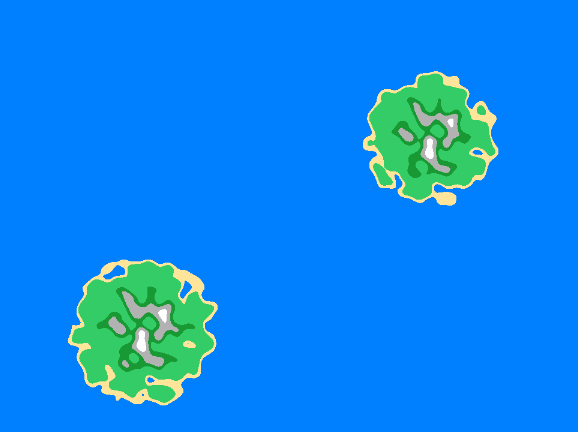# Problem with generating islands (SOLVED)

#1

Hello, i have a stabbing problem. I tried to speed up the process of generating islands by using a larger number of smaller atlases. Of course, I try to generate different objects, but when I create a second object with an atlas to generate an island, the first island is automatically drawn on it without adding a script to the new object. The same thing happens when I change the name of an object and an atlas. Don’t know where the mistake is?
And here is the script:

``````local perlin = require('main.perlin_noise')
local rgb = hash('rgb')

local colors = {
water = 255 * vmath.vector3(0.2, 0.5, 1),
sand = 255 * vmath.vector3(1, 0.9, 0.6),
grass = 255 * vmath.vector3(0.2, 0.8, 0.4),
forest = 255 * vmath.vector3(0.1, 0.6, 0.2),
rock = 255 * vmath.vector3(0.7, 0.7, 0.7),
snow = 255 * vmath.vector3(1, 1, 1)
}

local function set_pixel(stream, width, x, y, color)
local index = 3 * (x + y * width) + 1
stream[index] = color.x
stream[index + 1] = color.y
stream[index + 2] = color.z
end

function init(self)
local width = 256
local height = 256

self.buffer = buffer.create(width * height, {{name = rgb, type = buffer.VALUE_TYPE_UINT8, count = 3}})
local stream = buffer.get_stream(self.buffer, rgb)

local scale = 1 / 30
pos = vmath.vector3(128, 128, 0)
for x = 0, width - 1 do
for y = 0, height - 1 do
local v = 0.5 + perlin:noise(x * scale, y * scale, 0) / 2
distance = vmath.length(vmath.vector3(x, y, 0) - pos)
v = v - ((v/100) * part)
else v = 0 end

local color = colors.rock
if v < 0.1 then
color = colors.water
elseif v < 0.13 then
color = colors.sand
elseif v < 0.32 then
color = colors.grass
elseif v < 0.40 then
color = colors.forest
elseif v > 0.5 then
color = colors.snow
end
set_pixel(stream, width, x, y, color)
end
end

local resource_path = go.get('#sprite', 'texture0')
local header = {width = width, height = height, type = resource.TEXTURE_TYPE_2D, format = resource.TEXTURE_FORMAT_RGB, num_mip_maps = 1}
end``````
0 Likes

#2
``````	local resource_path = go.get('#sprite', 'texture0')
local header = {width = width, height = height, type = resource.TEXTURE_TYPE_2D, format = resource.TEXTURE_FORMAT_RGB, num_mip_maps = 1}
``````

It looks like the resource they all share might be being overwritten by each other? You could test this by having 2 island with 2 unique starter atlases associated with their sprite. But there is probably a better way to deal with this. If you just want to generate several islands in advance you could have like 5 generated at once each associated with one atlas and then cycle through them / their sprite id.

0 Likes

#3

Thank you very much for the answer, unfortunately it still doesn’t help, although I assign different atlases to objects, the result is still bad.

0 Likes

#4

Please make and post a minimal project where it works not as you expect. I’m going to make a test and see if it is not working how I would expect it to.

0 Likes

#5

MultiResourceBufferSprites.zip (7.2 KB)

Here is a sample where 3 different objects get their textures changed as expected. So either something I don’t understand about the problem, or something else you are doing is making what you are doing breaking.

``````go.property("r", 255)
go.property("g", 255)
go.property("b", 255)

local function set_pixel(stream, width, x, y, color)
local index = 3 * (x + y * width) + 1
stream[index] = color.x
stream[index + 1] = color.y
stream[index + 2] = color.z
end

local function actual_init(self)
local width = 64
local height = 64
local rgb = hash("rgb")

local declaration = {{
name = rgb,
type = buffer.VALUE_TYPE_UINT8, count = 3
}}
local abuffer = buffer.create(width * height, declaration)
local stream = buffer.get_stream(abuffer, rgb)

for x = 0, width - 1 do
for y = 0, height - 1 do
local color = vmath.vector3(self.r, self.g, self.b)
set_pixel(stream, width, x, y, color)
end
end

local resource_path = go.get('#sprite', 'texture0')
local header = {width = width, height = height, type = resource.TEXTURE_TYPE_2D, format = resource.TEXTURE_FORMAT_RGB, num_mip_maps = 1}
end
``````

Also if you want resources to be generated faster you will probably want some of your game to be native code via native extension.

I also tested not having scripts on the other two

3 Likes

#6

@Pkeod, thank you very much for your answers. It’s not still working for me, so there I send you the project:

Noise.zip (31.5 KB)

0 Likes

#7

It looks like you need both a unique atlas and a unique texture within the unique atlas. Maybe it’s an optimization Defold does silently that messes this specific situation up.

0 Likes

#8

I was trying using unique atlas and texture too and Itwas the same.

0 Likes

#9Noise.zip (8.7 KB)

Here they are different now, you should be able to change the randomness of the noise to whatever you want.

There was also a problem with the script it was referencing the go, I changed it to

``````local resource_path = go.get('#sprite', 'texture0')
``````
1 Like

#10

That’s really great, thank you very much @Pkeod!

1 Like